# Mastermind

Published: 17/01/2016

Mastermind is a board game played in the following way:

• One player creates a codeword, consisting of an ordered sequence of four coloured counter, where each counter can take one of six colours. The codeword can contain any colour in any position, making $6^{4} = 1296$ possible codewords.
• A second player guesses the codeword.
• After the guess, the second player receives feedback in the form of a score. The score consists of up to four black and white markers. Each black marker means that the guess has a counter of the same colour, and the same position as the codeword. A white counter means that guess has the same coloured counter, but in a different position to the code word.
• If the second player didn't correctly guess the codeword, they make another guess.
• The game ends when the second player correctly guesses the code word. The goal is to do this in the smallest number of guesses possible.

It seems like a game ripe to be solved by a computer, and indeed it is. Knuth proved that any game is can be solved in five guesses or less.

Here, I'm going to implement a few different strategies for playing mastermind and compare them.

In :
#first, let's set up some details of the problem
from itertools import product

NUMBER_OF_SYMBOLS = 6
SYMBOLS = list(range(NUMBER_OF_SYMBOLS))
PATTERN_LENGTH = 4
ALL_POSSIBLE_VALUES = tuple(product(SYMBOLS, repeat=PATTERN_LENGTH))
POSSIBLE_SCORES = [(i,j) for i in range(5) for j in range(5)
if (i + j < 5) and not (i == 3 and j == 1)]


In what follows we use the integers 0-5 to represent the six colours, and a 4-tuple of these to represent the codeword or a guess.

With this in mind, we can write a function that scores a guess.

In :
#scoring
from collections import defaultdict

def score(guess, truth):
"""
Scoring for mastermind.

Returns a 2-tuple.
The first element is the number of symbols with the same
value, and in the same position in  "guess" and "truth".

The second is and the number of similar symbols, but with
different positions between "guess" and "truth", ignoring those
counted in the first element.
"""
assert(len(guess) == len(truth))

in_order = 0
out_of_order = 0
truth_ooo = defaultdict(int)
guess_ooo = defaultdict(int)

#check in order positions
for g,t in zip(guess, truth):
if g == t:
in_order += 1
else:
truth_ooo[t] += 1
guess_ooo[g] += 1

#check out of order positions
for k in guess_ooo.keys():
out_of_order += min(guess_ooo[k], truth_ooo[k])

return (in_order, out_of_order)


Once we have that, we can also define a function update_possible, which takes a list of possible codewords, a guess and the guesses score, and returns only those values which are consistent with the guess and score.

In :
def update_possible(possible_values, guess, guess_score):
"""
Returns the subset of possible_values the give guess_score
in response to guess.
"""
return tuple(value for value in possible_values
if score(guess, value) == guess_score)


Our solvers are going to take the form of a function that takes a list of possible codewords, and the guess number we're on, and returns a guess.

To test how good each of these solvers are, we are going to simulate them playing the game with the following functions

In :
import time
import matplotlib.pyplot as plt
%matplotlib inline
import matplotlib as mpl
mpl.style.use("ggplot")

import seaborn as sns

def run_solver(solver, initial_possible_values, true_value,
guess_limit=12, printit=False):
"""
Repeatedly applies solver to valid_values until either limit
is reach, or a single answer has been found. Returns the
number of guesses required, or -1 if guess_limit is exceeded.
"""
number_of_guesses = 0
valid_values = initial_possible_values[:]
t = time.time()

while(number_of_guesses <= guess_limit):
number_of_guesses += 1
current_guess = solver(valid_values, number_of_guesses)
current_score = score(current_guess, true_value)
valid_values = update_possible(valid_values, current_guess, current_score)

if printit:
print("Guess {0}, {1}".format(number_of_guesses, current_guess))
print("\tScore:{0}, number of valid values remaining: {1}, time taken: {2:.4f}"
.format(current_score, len(valid_values), time.time() - t))
t = time.time()

#check for success
if current_score == PATTERN_LENGTH:
if printit:
print("Success!")
return number_of_guesses

return -1

def evaluate_solver(solver, initial_possible_values, possible_truth_values,
printit=False):
"""
Applies solver to each possible_truth_value, returning an list of
how many guesses it takes for each to solve.
"""
t = time.time()
time_record = []
guess_record = []

for truth in possible_truth_values:
guess_record.append(run_solver(solver, initial_possible_values, truth))
time_record.append(time.time()-t)
t = time.time()

if printit:
not_exceeded_limit = [g for g in guess_record if g > -1]
N = len(possible_truth_values)
print(solver.__name__)
print("----------")
print("Average number of guesses: {0:.4f}".format(sum(not_exceeded_limit)
/ len(not_exceeded_limit)))
print("Max number of guesses: {0}".format(max(not_exceeded_limit)))
print("Number of guess limits exceeded: {0}".format(N - len(not_exceeded_limit)))
print("Average time taken per run: {0:.4f}".format(sum(time_record)/N))
plt.hist(not_exceeded_limit, bins=range(1,13))
plt.xticks([x+0.5 for x in range(1,13)], range(1,13))
plt.xlim(1,13)
plt.title("Distribution of number of guesses required to find the codeword")

return guess_record


## Solvers that Guess¶

We can now implement the simplest solver I can think of: one that just chooses a guess at random from the available consistent values.

In :
import random

def random_consistant_solver(possible_values, number_of_guesses):
"""
Guesses a random Value from possible_values
"""
return random.choice(possible_values)

In :
evaluate_solver(random_consistant_solver, ALL_POSSIBLE_VALUES,
ALL_POSSIBLE_VALUES, printit=True);

random_consistant_solver
----------
Average number of guesses: 4.6003
Max number of guesses: 7
Number of guess limits exceeded: 0
Average time taken per run: 0.0068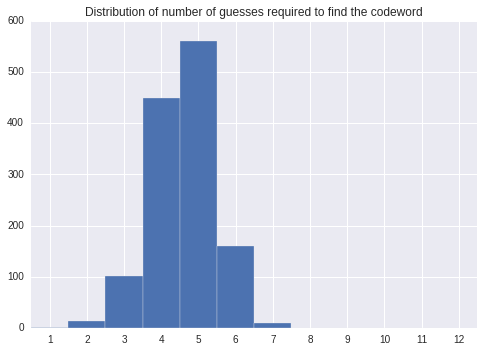The random guessing solver isn't bad. It's pretty close to how I would play the game.

But is there a better way? Intuition tells me that rather than guessing a consistent value at random, we might do better by choosing the value which reduces the number of possible values the most. If we knew the true value, this would be trivial: we just guess the true value and our number of possible values is reduced to one. But we don't, so we have to consider the effect of our guess on all possible values.

There are two ways of doing this. The first is to guess the value that returns the greatest reduction, when averaged across all possible values (I'll call this "best_expected_solver"). Another way is to guess the value that returns the greatest reduction, in the worst possible case, when averaged across the possible values (I'll call this "best_worst_case_solver").

Carrying out these calculations isn't easy though. Updating our possible values given a guess is $O(N)$, for $N$ possible values. Averaging over all possible values for a given guess is then $O(N^{2})$, and minimising over all possible guesses is $O(N^{3})$. And this is only to calculate one guess.

This complexity is most apparent on the first guess of the game, when the set of possible values is largest. However, we can reduce it using the fact that we are assuming the true value is drawn uniformly from all possible values. A first guess like (1,1,1,1) gives us as much information about the true value as a first guess like (0,0,0,0) when averaged across all possible values. The same is true of the two guesses (1,0,0,0) and (0,1,0,0). This is a reflection of the fact that the problem doesn't change if we re-label the symbols or if we permute the order of the symbols.

Because of this there are only really five guesses worth starting with:

In :
STARTING_GUESSES = [(0,0,0,0), (1,0,0,0), (1,1,0,0), (1,2,0,0), (0,1,2,3)]


A further optimization we can make is for best_worst_case_solver. Because we are only looking for the worst case for each guess, rather than averaging over all possible values, we can average over all possible hints. There are only 15 possible hints (hint (3,1) is not possible).

In :
def best_expected_solver(possible_values, number_of_guesses):
"""
Returns the guess which reduces the number of possible values
the most, when averaged across all possible_values.
"""
best_guess = possible_values
best_score = 10**6

if number_of_guesses == 1:
possible_guess_values = STARTING_GUESSES
else:
possible_guess_values = possible_values

for guess in possible_guess_values:
remaining_lengths = []
for possible_truth in possible_values:
inner_score = score(guess, possible_truth)
updated_possible_values = update_possible(possible_values, guess, inner_score)
remaining_lengths.append(len(updated_possible_values))

guess_score = sum(remaining_lengths) / len(remaining_lengths)
if guess_score < best_score:
best_score = guess_score
best_guess = guess

return best_guess

def best_worst_case_solver(possible_values, number_of_guesses):
"""
Returns the guess which reduces the number of possible values
the most, when considering the worst guess in possible_values.
"""
best_guess = possible_values
best_score = 10**6

if number_of_guesses == 1:
possible_guess_values = STARTING_GUESSES
else:
possible_guess_values = possible_values

for guess in possible_guess_values:
remaining_lengths = []
for inner_score in POSSIBLE_SCORES:
updated_possible_values = update_possible(possible_values, guess, inner_score)
if len(updated_possible_values) > 0:
remaining_lengths.append(len(updated_possible_values))

guess_score = max(remaining_lengths)
if guess_score < best_score:
best_score = guess_score
best_guess = guess

return best_guess


Let's test them both.

In :
truth = random.choice(ALL_POSSIBLE_VALUES)
print("Codeword is: {}\n".format(truth))
run_solver(best_expected_solver, ALL_POSSIBLE_VALUES, truth , printit=True);

Codeword is: (2, 1, 3, 2)

Guess 1, (1, 2, 0, 0)
Score:(0, 2), number of valid values remaining: 222, time taken: 34.6978
Guess 2, (2, 1, 3, 4)
Score:(3, 0), number of valid values remaining: 10, time taken: 47.8837
Guess 3, (2, 1, 1, 4)
Score:(2, 0), number of valid values remaining: 5, time taken: 0.0040
Guess 4, (2, 1, 3, 2)
Score:(4, 0), number of valid values remaining: 1, time taken: 0.0006
Success!

In :
truth = random.choice(ALL_POSSIBLE_VALUES)
print("Codeword is: {}\n".format(truth))
run_solver(best_worst_case_solver, ALL_POSSIBLE_VALUES, truth, printit=True);

Codeword is: (5, 5, 5, 0)

Guess 1, (1, 1, 0, 0)
Score:(1, 0), number of valid values remaining: 256, time taken: 0.3841
Guess 2, (1, 2, 3, 3)
Score:(0, 0), number of valid values remaining: 16, time taken: 4.0286
Guess 3, (4, 4, 0, 4)
Score:(0, 1), number of valid values remaining: 1, time taken: 0.0141
Guess 4, (5, 5, 5, 0)
Score:(4, 0), number of valid values remaining: 1, time taken: 0.0001
Success!


The functions are taking long enough that testing them over all possible values will take too long to test easily. However, since each function is deterministic, we can memorize the outputs and avoid some unnecessary computations.

In :
class Memorize:
"""
A class to memorize the output of a function, based only on the first argument.
"""

def __init__(self, func):
self.memory = {}
self.func = func
self.__name__ = func.__name__ + "_memorized"

def __call__(self, *input_values):
if input_values in self.memory:
return self.memory[input_values]
result = self.func(*input_values)
self.memory[input_values] = result
return result

def get_memory(self):
return self.memory

best_expected_solver_memorized = Memorize(best_expected_solver)
best_worst_case_solver_memorized = Memorize(best_worst_case_solver)


We can now test them over all possible inputs

In :
%time scores = evaluate_solver(best_expected_solver_memorized, \
ALL_POSSIBLE_VALUES, ALL_POSSIBLE_VALUES, printit=True);

best_expected_solver_memorized
----------
Average number of guesses: 4.4144
Max number of guesses: 6
Number of guess limits exceeded: 0
Average time taken per run: 62.0130
CPU times: user 4min 42s, sys: 52 ms, total: 4min 42s
Wall time: 22h 19min 28s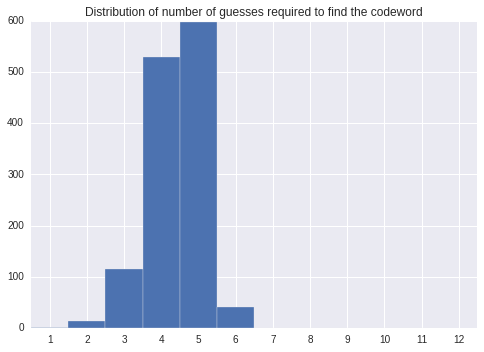In :
%time scores = evaluate_solver(best_worst_case_solver_memorized, \
ALL_POSSIBLE_VALUES, ALL_POSSIBLE_VALUES, printit=True);

best_worst_case_solver_memorized
----------
Average number of guesses: 4.5000
Max number of guesses: 6
Number of guess limits exceeded: 0
Average time taken per run: 0.0235
CPU times: user 30.5 s, sys: 48 ms, total: 30.5 s
Wall time: 30.5 s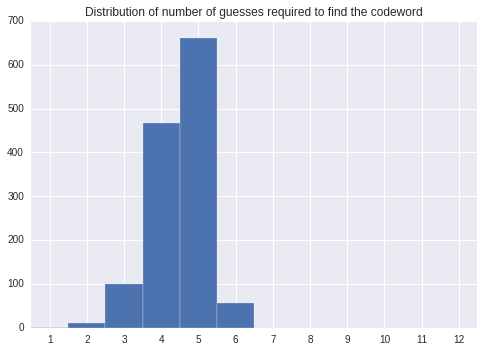Unsuprisingly, best_expected_solver beats the others on average, and best_worst_case_solver reduces the number of guesses required. But if you remember back to the start, I said that it was possible to solve the game in five moves or fewer.

The difference lies in what guesses the solver can make. Consider the following game:

In :
truth = (1, 5, 0, 0)
print("Codeword is: {}\n".format(truth))
run_solver(best_worst_case_solver_memorized, ALL_POSSIBLE_VALUES, truth, printit=True);

Codeword is: (1, 5, 0, 0)

Guess 1, (1, 1, 0, 0)
Score:(3, 0), number of valid values remaining: 20, time taken: 0.0112
Guess 2, (1, 1, 0, 2)
Score:(2, 0), number of valid values remaining: 8, time taken: 0.0002
Guess 3, (0, 1, 0, 0)
Score:(2, 1), number of valid values remaining: 3, time taken: 0.0001
Guess 4, (1, 3, 0, 0)
Score:(3, 0), number of valid values remaining: 2, time taken: 0.0000
Guess 5, (1, 4, 0, 0)
Score:(3, 0), number of valid values remaining: 1, time taken: 0.0000
Guess 6, (1, 5, 0, 0)
Score:(4, 0), number of valid values remaining: 1, time taken: 0.0000
Success!


After the first guess, we have 20 remaining possible_values. Guessing (1,1,0,2) will reduce this to eight, but if we guess (0,1,0,2), we reduce this to five possible values. However, (0,1,0,2) isn't actually in the set of possible values after our first guess.

## Knuth's solution¶

As noted in Knuth’s paper, situations can arise where guessing a value that could win on the next go is not always the best strategy. To incorporate this change, we can replace the loop in the previous solution over all remaining possible_values with one over ALL_POSSIBLE_VALUES.

In :
def tie_breaker_lowest(guess1, guess2):
"""
Returns True if guess1 is numberically lower than guess2,
false if higher or identical.
"""
for x,y in zip(guess1, guess2):
if x < y:
return True
elif x > y:
return False
return False

def knuth_solver(possible_values, number_of_guesses,
tie_breaker=tie_breaker_lowest):
"""
A solver based on Knuth's paper.
"""
best_guess = possible_values
best_score = 10**6

if number_of_guesses == 1:
possible_guess_values = STARTING_GUESSES
else:
possible_guess_values = ALL_POSSIBLE_VALUES

possible_guess_set = set(possible_values)

for guess in possible_guess_values:
remaining_lengths = []
for inner_score in POSSIBLE_SCORES:
updated_possible_values = update_possible(possible_values, guess, inner_score)
remaining_lengths.append(len(updated_possible_values))

guess_score = max(remaining_lengths)

if guess_score < best_score:
best_score = guess_score
best_guess = guess
# in case of ties, always pick a guess in possibe_values
# if both guesses are in possible_values, use the function
# tie_breaker.
elif guess_score == best_score:
if guess in possible_guess_set and best_guess in possible_guess_set:
if tie_breaker(guess, best_guess):
best_score = guess_score
best_guess = guess
elif guess in possible_guess_set:
best_score = guess_score
best_guess = guess

return best_guess

knuth_solver_memorized = Memorize(knuth_solver)

In :
%time scores = evaluate_solver(knuth_solver_memorized, \
ALL_POSSIBLE_VALUES, ALL_POSSIBLE_VALUES, printit=True);

knuth_solver_memorized
----------
Average number of guesses: 4.4738
Max number of guesses: 5
Number of guess limits exceeded: 0
Average time taken per run: 0.3385
CPU times: user 7min 18s, sys: 16 ms, total: 7min 18s
Wall time: 7min 18s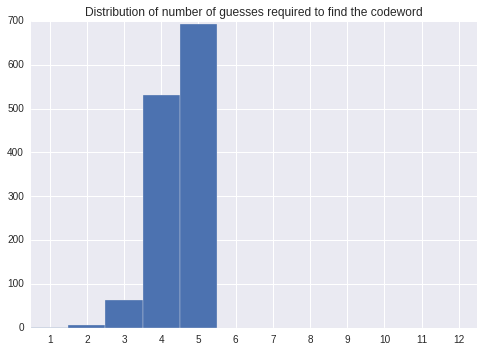And we recover the results from Knuth's paper - a strategy that can always find the solution in five moves or less.

I noticed something interesting when writing this solver: the order in which you resolve ties makes a difference. When two possible guesses result in the same worst case length of possible solution, we choose the one that lies in possible_values. When both guesses lie in possible_values, Knuth chooses the one with the lowest numerical value. If you happen to choose the one with the highest value, you can't always solve the problem in five or fewer moves, as shown below:

In :
def tie_breaker_highest(guess1, guess2):
"""
Returns True if guess1 is numberically Higher than guess2,
False if lower or identical.
"""
for x,y in zip(guess1, guess2):
if x > y:
return True
elif x < y:
return False
return False

knuth_solver_memorized2 = Memorize(lambda i,j : knuth_solver(i,j, tie_breaker = tie_breaker_highest))

%time scores = evaluate_solver(knuth_solver_memorized2, \
ALL_POSSIBLE_VALUES, ALL_POSSIBLE_VALUES, printit=True);

<lambda>_memorized
----------
Average number of guesses: 4.4745
Max number of guesses: 6
Number of guess limits exceeded: 0
Average time taken per run: 0.3400
CPU times: user 7min 19s, sys: 544 ms, total: 7min 20s
Wall time: 7min 20s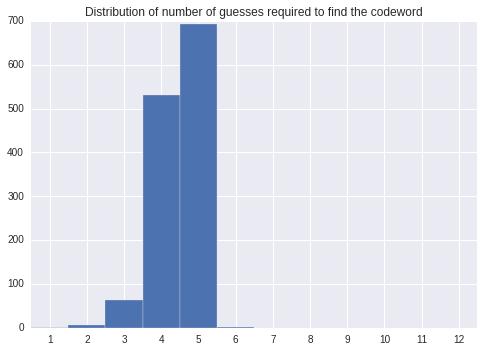# This Post¶

This post was written as an ipython notebook that can be found here.